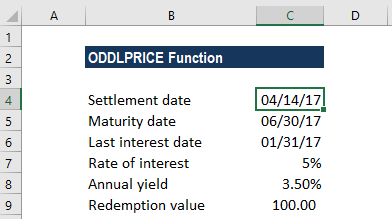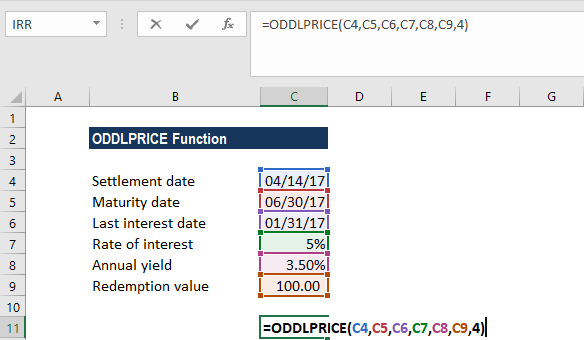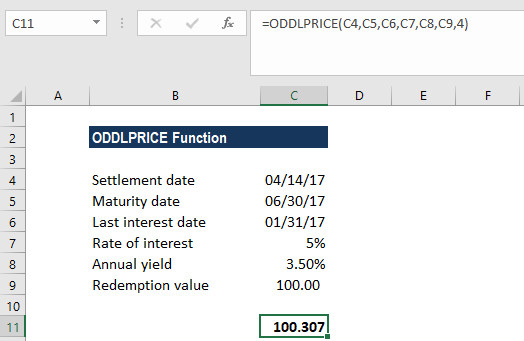# ODDLPRICE Function

Calculates the price per \$100 face value of a security with an odd last period

## What is the ODDLPRICE Function?

The ODDLPRICE function is categorized under Excel Financial functions. It helps calculate the price per \$100 face value of a security with an odd last period.

In financial analysis, some bonds come with irregular first or last periods. Due to the irregular first or last period, the payment doesn’t fit in any of the given patterns. If we wish to calculate the price of a bond with an odd last period, we can use the ODDLPRICE function.

### Formula

=ODDLPRICE(settlement, maturity, last_interest, rate, yld, redemption, frequency, [basis])

The ODDLPRICE function uses the following arguments:

1. Settlement (required argument) – This is the security’s settlement date. it is the date after the issue date when the security is traded to the buyer.
2. Maturity (required argument) – This is the security’s maturity date. It is the date when the security expires.
3. Last_interest (required argument) – This is the security’s last coupon.
4. Rate (required argument) – The security’s interest rate.
5. Yld (required argument) – The security’s annual yield.
6. Redemption (required argument) – This is the security’s redemption value per \$100 face value.
7. Frequency (required argument) – This is the number of coupon payments per year. For annual payments, frequency = 1; for semiannual, frequency = 2; for quarterly, frequency = 4.
8. Basis (optional argument) – It is the type of day count basis to use. The possible values of the basis are as follows:

BasisDay Count basis
0 or omittedUS(NASD) 30/360
1Actual/actual
2Actual/360
3Actual/365
4European 30/360

### How to use the ODDLPRICE Function in Excel?

To understand the uses of the ODDLPRICE function, let’s consider an example:

#### Example

Let’s assume we need to calculate the price per \$100 face value of a security with the last interest date as Jan. 31, 2017, a settlement date of Apr. 14, 2017, and a maturity date Jun. 30, 2017. The rate of interest is 5%, the annual yield is 3.5%, and the redemption value is \$100. Payments are made quarterly and the US (NASD) 30/360 day count basis is used:The formula used is:We get the result below:The above function returned the value 100.307. That is, the security with the above terms would be valued at \$100.307. In the example:

• The date arguments were supplied to the ODDLPRICE function as references to cells containing dates.
• The rate and yield arguments were entered as percentages, 5% and 3.5%, respectively. However, the arguments could, instead, be entered as the simple numeric values 0.005 and 0.035, respectively.
• As we omitted the [basis] argument, the function uses the default value 0, which denotes the US (NADS) 30/360 day count basis.

### Notes about the ODDLPRICE Function

1. #NUM! error – Occurs when:
• The given issue date is greater than or equal to the settlement date.
• The given settlement date is greater than or equal to the maturity date.
• We provided invalid numbers for the rate, yield, redemption, frequency or [basis] arguments. That is if either rate is less than 0; yield is less than 0; redemption is less than or equal to 0; frequency is any number other than 1, 2, or 4; or [basis] is any number other than 0, 1, 2, 3, or 4).
2. #VALUE! error – Occurs when:
• The given settlement, maturity, last_interest arguments are not valid Excel dates.
• Any of the given arguments is non-numeric.

Thanks for reading CFI’s guide to the Excel ODDLPRICE function. By taking the time to learn and master these functions, you’ll significantly speed up your financial analysis. To learn more, check out these additional CFI resources:

• Excel Functions for Finance